Next: Worked example 1.3: Dimensional Up: Introduction Previous: Worked example 1.1: Conversion

## Worked example 1.2: Tire pressure

Question: The recommended tire pressure in a Honda Civic is 28 psi (pounds per square inch). What is this pressure in atmospheres (1 atmosphere is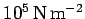)?

Answer: First, 28 pounds per square inch is the same as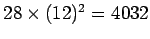pounds per square foot (the standard fps unit of pressure). Now, 1 pound equals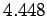Newtons (the standard SI unit of force), and 1 foot equals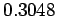m (see Tab. 2). Hence,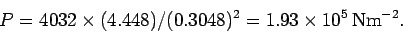It follows that 28 psi is equivalent to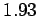atmospheres.

Richard Fitzpatrick 2006-02-02